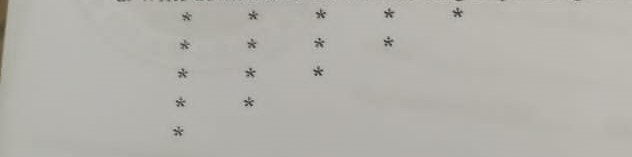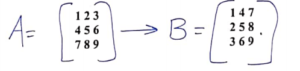# Programming fundamental  all previous/ past question papers

– Partial Differential Equations –Computer Science all subject past paper Partial Differential Equations-Computer Graphics  Course  Partial Differential Equations Project idea – ICT  MCQ -Partial Differential Equations Interview Question  –Computer Science all courses -Technology –University Past Paper -Programming language –Question paperold paper

Q1:

1. Write down the difference between compiler and interpreter?
2. Write down difference between operator precedence and operator associativity in C++?
3. Write down the code for the following output using for loop?Q2: Write a program that repeatedly collects positive integers from the user, stopping when the user enters a negative number or zero. After that, output the product of all positive entries?

Q3: Input three integer numbers and find the middle number of three integers.

Hint1: 23 54 35                  Hint2: 40 23 50                  Hint3: 34 54 65

Middle No is: 35                Middle No is: 40               Middle No is: 54

Q4: Write a program in C++ to display the number’s digits in reverse order?

Sample output:

Input a number: 12345

The number in reverse order is: 54321

## Postcolonial Literature Sessional I in past paper

ATTEMPT ALL QUESTIONS.

Q1

Write a program that inputs radius from the user and calculates area and circumference of a circle by using the formula Area- pix (radius)² & Circumference = 2 x pi x radius (3)

Q-2

Draw flowchart for a program that inputs three integer numbers and displays the smallest number by using nested if structure.

Q-3

a) Write a code with flowchart that finds the sum of the squares of integers from I to 100.

b) Compare if, if-else-if and nested if structures(3)

## Postcolonial Literature Sessional II in past paper

Q1

Write a program that inputs five integers in an array and passes array to a function. The function counts total number of even and odd numbers and returns to main function to display it(10)

Q-2 Write a program that inputs values in a two dimensional array with three rows and two columns, The function returns the maximum value in the array. (5)

Q-3 Write a program for matrix A that inputs following elements & arrange these elements shown in matrix B as under:–.Q-4 Write a program that inputs ten floating point numbers in an array. It displays the values which are greater or equal or less than average value of this array. (10)

## Postcolonial Literature Final paper

Q-1: Study the following code segments and write the output [2.5° 2-5).

int N = 7; int i, x;

for (= 1; i <= N; i++) {

cin >> x; cout <<<< “, ” << X << ” “; if(1 % 3= 0) { cout << endl; }

int arr-{0,1,0,1,1); int sum-0,p=0; for (int i=0;i<5;i++){ sam-sum+pow(2.p) arr;

cout << endl what is displayed with the given input? watch the end 1 characters.

p++;

}

cout<<sum<<<endl;

Input: 11 12 13 14 15 16 17 18 19

Q-2: Rewrite the following C++ code segments to make it more efficient and to remove any unnecessary code. [2.5* 2-5).

if (x <yly >= x) { cout <<

“Not good. \n”; }

cout << “Carry on.\n”;

if (x / y != 0 && y != 0) { cout << x/y << endl; }

Q-3: Write a C++ program to find k largest elements in a given array of integers. 

Data Structure and Algorithms

1. Write an algorithm to delete even valued elements from an integer type array,
2. Suppose an array A containing ten integers as shown, Draw the resultant array after implementing the given loop in each part. (In part-b use the given array, not the resultant array of part-a)
 10 20 30 40 50 60 70 80 90 100

 Part-a Part-b Repeat for kß1 to 9 A[K+1] ß A[K] Kß 9 Repeat while (K>=1) A[K+1] ß A Kß K-1

iii. Using binary search technique show how it works when searching for 17 in the

following array. (Assume indexing starts from 1 to 9)

 1 5 6 9 10 12 14 17 21

Question No. 2: Write short answers of questions given bellow:

1. What are the types of asymptotic notations?
2. Which asymptotic notations specifically describes best and average case scenarios?

Write difference between traversing and searching

1. Define the situations when insertion in an array becomes best and worst.